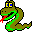#Boost C++ Libraries

...one of the most highly regarded and expertly designed C++ library projects in the world.#isomorphism

```// named parameter version
template <class Graph1, class Graph2, class P, class T, class R>
bool isomorphism(const Graph1& g1, const Graph2& g2,
const bgl_named_params<P,T,R>& params = all defaults)

// non-named parameter version
template <typename Graph1, typename Graph2, typename IsoMap,
typename VertexInvariant1, typename VertexInvariant2,
typename V1Map, typename V2Map>
bool isomorphism(const Graph1& g1, const Graph2& g2,
IsoMap f, VertexInvariant1 invariant2, VertexInvariant2 invariant2,
std::size_t max_invariant, VertexIndex1Map i1_map, VertexIndex2Map i2_map)
```

An isomorphism is a 1-to-1 mapping of the vertices in one graph to the vertices of another graph such that adjacency is preserved. Another words, given graphs G1 = (V1,E1) and G2 = (V2,E2) an isomorphism is a function f such that for all pairs of vertices a,b in V1, edge (a,b) is in E1 if and only if edge (f(a),f(b)) is in E2.

This function returns true if there exists an isomorphism between graph 1 and graph 2 and false otherwise. Also, if a isomorphism map named parameter is provided then an isomorphism is recorded in the map.

The current implementation is based on descriptions of a backtracking algorithm in [46,48]. The file isomorphism-impl.pdf contains a "literate" description of the implementation. The algorithm used is simple but slow. A more efficient (and much more complex) algorithm is described in . When (and if) a version of this algorithm is ported to the BGL interface it should replace the current algorithm.

### Where Defined

boost/graph/isomorphism.hpp

### Parameters

IN: const Graph1& g1
A directed or undirected graph. The graph type must model of Vertex List Graph and Edge List Graph.
IN: const Graph2& g2
A directed or undirected graph. The graph type must model Bidirectional Graph and Vertex List Graph.

### Named Parameters

OUT: isomorphism_map(IsoMap f)
The mapping from vertices in graph 1 to vertices in graph 2. This must be a Read/Write Property Map.
Default: an iterator_property_map constructed from a std::vector of graph 2's vertex descriptor type and the vertex index map for graph 1.
Python: Must be a vertex_vertex_map for the first graph.
IN: vertex_invariant1(VertexInvariant1 i)
A mapping i from vertices to integers such that if there is some isomorphism that maps v onto v' then i(v) == i(v'). The VertexInvariant type must be a BinaryFunction where the first argument is a vertex descriptor, the second argument is a graph, and the result type is an integer. The vertex invariant must work with the types for graph 1.
Default: degree_vertex_invariant
Python: Unsupported parameter.
IN: vertex_invariant2(VertexInvariant2 i)
A mapping i from vertices to integers such that if there is some isomorphism that maps v onto v' then i(v) == i(v'). The VertexInvariant type must be a BinaryFunction where the first argument is a vertex descriptor, the second argument is a graph, and the result type is an integer. The vertex invariant must work with the types for both graph 2.
Default: degree_vertex_invariant
Python: Unsupported parameter.
IN: vertex_max_invariant(std::size_t max_invariant)
An upper bound on the possible values returned from either vertex_invariant1 or vertex_invariant2.
Default: vertex_invariant2.max(). The default vertex_invariant2 parameter, an instance of degree_vertex_invariant, defines this function to return num_vertices(g2) * (num_vertices(g2)+1).
Python: Unsupported parameter.
IN: vertex_index1_map(VertexIndex1Map i1_map)
This maps each vertex to an integer in the range [0, num_vertices(g)). This is necessary for efficient updates of the heap data structure when an edge is relaxed. The type VertexIndex1Map must be a model of Readable Property Map. The value type of the map must be an integer type. The vertex descriptor type of graph 1 needs to be usable as the key type of the map.
Default: get(vertex_index, g1) Note: if you use this default, make sure your graph has an internal vertex_index property. For example, adjacenty_list with VertexList=listS does not have an internal vertex_index property.
Python: Unsupported parameter.
IN: vertex_index2_map(VertexIndex2Map i2_map)
This maps each vertex to an integer in the range [0, num_vertices(g)). This is necessary for efficient updates of the heap data structure when an edge is relaxed. The type VertexIndex2Map must be a model of Readable Property Map. The value type of the map must be an integer type. The vertex descriptor type of graph 2 needs to be usable as the key type of the map.
Default: get(vertex_index, g2) Note: if you use this default, make sure your graph has an internal vertex_index property. For example, adjacenty_list with VertexList=listS does not have an internal vertex_index property.
Python: Unsupported parameter.

### Complexity

The worst-case time complexity is O(|V|!).

### Example

See libs/graph/example/isomorphism.cpp.